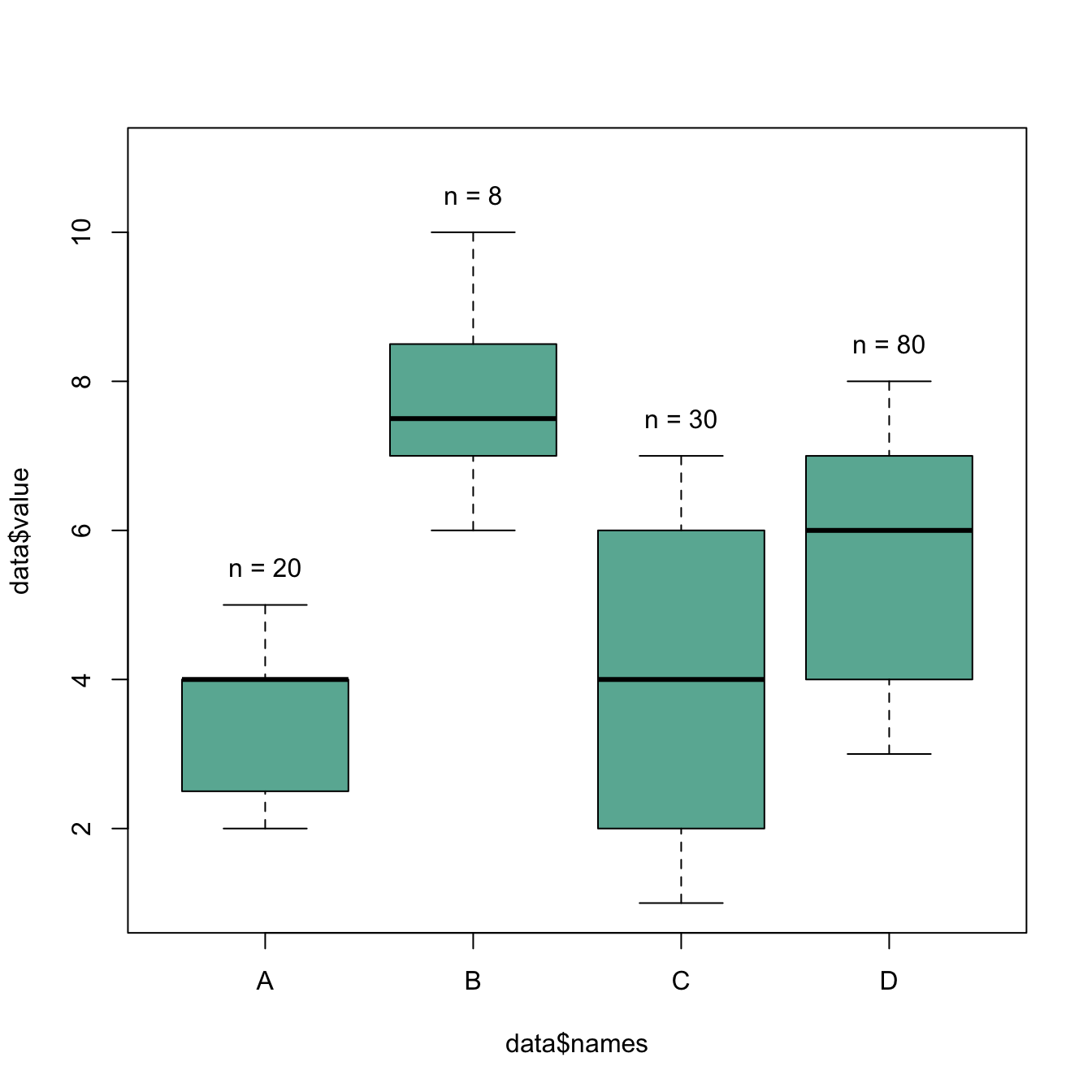# Add text over boxplot in base R

This examples demonstrates how to build a boxplot with sample size written on top of each box. It is useful to indicate what sample size is hidden behind each box. Basic R implementation.

The first challenge here is to recover the position of the top part of each box. This is done by saving the `boxplot()` result in an object (called `boundaries` here). Now, typing `boundaries\$stats` gives a dataframe with all information concerning boxes.

Then, it is possible to use the `text` function to add labels on top of each box. This function takes 3 inputs:

• x axis positions of the labels. In our case, it will be 1,2,3,4 for 4 boxes.
• y axis positions, available in the `boundaries\$stats` object.
• text of the labels : the number of value per group or whatever else.``````# Dummy data
names <- c(rep("A", 20) , rep("B", 8) , rep("C", 30), rep("D", 80))
value <- c( sample(2:5, 20 , replace=T) , sample(4:10, 8 , replace=T),
sample(1:7, 30 , replace=T), sample(3:8, 80 , replace=T) )
data <- data.frame(names,value)

# Draw the boxplot. Note result is also stored in a object called boundaries
boundaries <- boxplot(data\$value ~ data\$names , col="#69b3a2" , ylim=c(1,11))
# Now you can type boundaries\$stats to get the boundaries of the boxes

# Add sample size on top
nbGroup <- nlevels(data\$names)
text(
x=c(1:nbGroup),
y=boundaries\$stats[nrow(boundaries\$stats),] + 0.5,
paste("n = ",table(data\$names),sep="")
)``````

Related chart types

## Contact

This document is a work by Yan Holtz. Any feedback is highly encouraged. You can fill an issue on Github, drop me a message on Twitter, or send an email pasting yan.holtz.data with gmail.com.